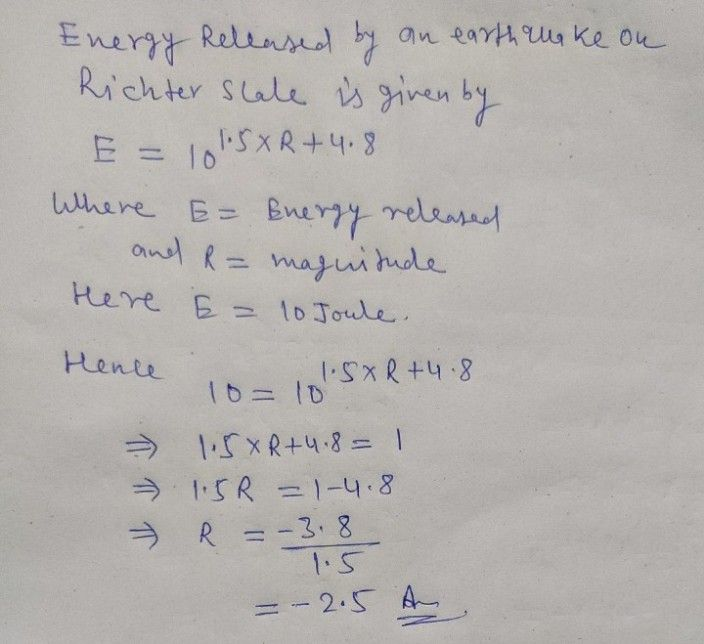Symbol
Problem$2$ Suppose that an earthquake released approximately $10^{6}$ joules $6$ ene what is the magnitude on a Richter scale? How much more energy does earthquake release than the reference earthquake?